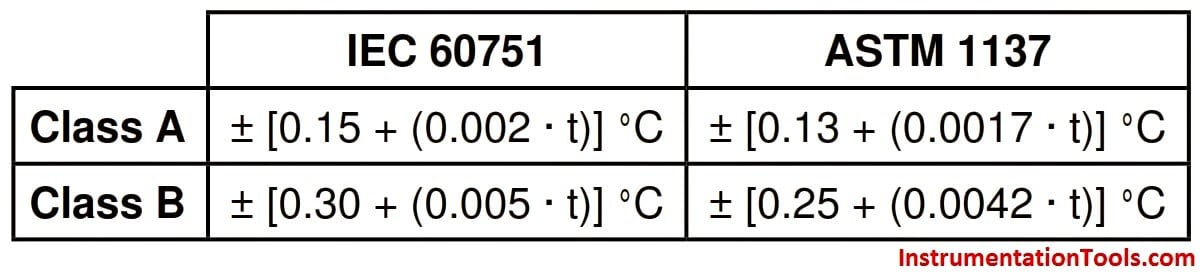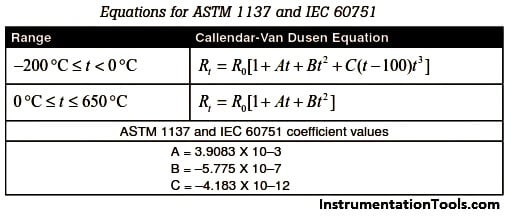# How to calculate the tolerance of a RTD sensor

The European standard, also known as the DIN or IEC standard, is considered the world-wide standard for Pt100. This standard, DIN/IEC 60751 (or simply IEC751), requires the RTD to have an electrical resistance of 100.00 Ω at 0°C and a temperature coefficient of resistance (alpha) of 0.00385 Ω/Ω/°C between 0 and 100°C.There are actually four resistance tolerances specified in DIN/IEC751:2008

Class AA = ± ( 0.10 + 0.0017 * | t | ) °C

Class A = ± ( 0.15 + 0.0020 * | t | ) °C

Class B = ± ( 0.30 + 0.0050 * | t | ) °C

Class C = ± ( 0.60 + 0.0100 * | t | ) °C

The combination of resistance tolerance and temperature coefficient define the resistance vs. temperature characteristics for the RTD sensor. The larger the element tolerance, the more the sensor will deviate from a generalized curve, and the more variation there will be from sensor to sensor (interchangeability). This is important for users who need to change or replace sensors and want to minimize interchangeability errors.

Tolerance Testing Method

RTD calibrations involving tolerance testing are reserved for low accuracy applications. The values are defined by one of the common models such as the ASTM 1137 or IEC 60751 curve. RTDs calibrated in this way are generally used in industrial style applications

The two common accuracy classes are class A and class B:These include errors arising from deviations in R0 and from errors in slope. Frequently, we will see probes rated at a fraction of Class A.

For example, 0.1 ASTM Class A. Fractional accuracy is achievable in sensors alone, but are very difficult to achieve in probes. The calculations are straightforward.Example: Calculate the accuracy of a 0.1 ASTM Class A probe at 100 °C

For ASTM standard:

Class A = ± [0.13 + (0.0017 · t)] °C
= (0.13 + (0.0017 · t)) · 0.1
= (0.13 + (0.0017 · 100)) · 0.1
= (0.13 + 0.17) · 0.1
= 0.03

Measure the reference probe temperature = 100.0 °C

Measure the indicated temperature = 100.5 °C

Calculate the error (Difference of Indicated & Reference) = 0.05 °C

Calculate the tolerance at 100.00 °C using ASTM standard = 0.03 °C

Determine tolerance status = Fail (0.05 °C > 0.03 °C)

The above example calculates the RTD tolerance at reference and indicated temperatures and determines the tolerance status of an RTD.

Be the first to get exclusive content straight to your email.
We promise not to spam you. You can unsubscribe at any time.

### 3 thoughts on “How to calculate the tolerance of a RTD sensor”

1.Hi,
This is a nice explanation. Can you make a tool for RTD tolerance calculation.
Regards,
Giri

•Hi Giri,

Thanks for the suggestion. Soon i will post a tool on RTD tolerance.
Thanks,
2.